Problem

# The uniform slender rod has a mass m. If it is released from rest when θ = 0°, determine t...

The uniform slender rod has a mass m. If it is released from rest when θ = 0°, determine the magnitude of the reactive force exerted on it by pin B whenθ= 90°.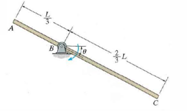#### Step-by-Step Solution

Solution 1

Draw the schematic diagram of the rod as shown below: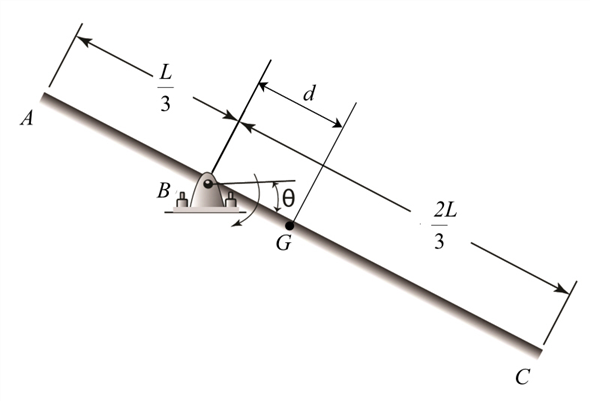Here, the centroid of the rod is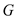.

Draw the free body diagram of the rod at any general position as shown below: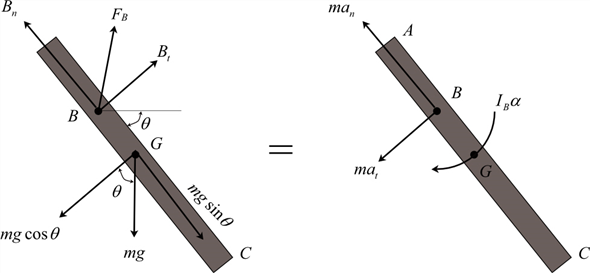Calculate the distance from the centroid of the rod to point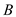as shown below.…… (1)

Here, the total length of the rod is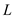and the distance from the centroid of the rod to pointis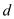.

Calculate moment of inertia of the rod about point B using the relation as shown below,…… (2)

Here, the mass of the rod is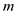, the moment of inertia of the rod about B is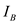and the moment of inertia of the rod about the centroid is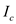.

Substitute equation (1) in equation (2).…… (3)

Consider the equilibrium of moments about the point B.…… (4)

Here, the angular acceleration is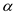and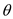is the inclination of rod from the horizontal in clockwise direction.

Substitute equation (1) and equation (3) in equation (4).…… (5)

Calculate the angular velocity of the rod at any general position. Consider the following kinematic equation.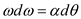…… (6)

Here,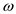is the angular velocity of the rod.

Substitute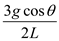forand integrate it.…… (7)

Consider the free body diagram of the rod. Write the equilibrium of forces along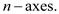…… (8)

Here, the force acting at pointalong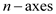is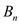.

Substitute equation (7) in equation (8).…… (9)

Substitute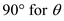in the above equation.Consider the equilibrium of forces along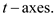…… (10)

Here, the force acting at pointalong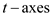is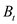.

Substitute equation (5) in equation (10).…… (11)

Substitutein the above equation.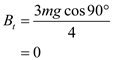Calculate the magnitude of reactive force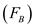acting on the rod by using the equation,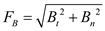Substitute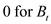and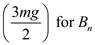.Hence, the magnitude of reactive forceacting on the rod is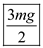.